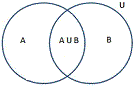# Union of Set(A Union B) or AUB Calculation

Set is the relation of some given data and has functions such as union and intersection.

 Enter the Set A: Enter the Set B:

Union of sets A and B(AUB) :

The term union means it is a collection of sets containing distinct elements. It is denoted by ∪.

#### Example:

The two sets of events A={1, 2, 3} and B={3, 4, 6, 7, 8}

these events are combined then we get :

A U B = {1, 2, 3, 4, 6, 7, 8}

### Definition

The union of set A and set B is represented by A U B and it is pronounced as A union B.

The union set contains the elements that belong to either A or to B or to both. The element is written only once even if they exist in both the sets

### Formula

n(A ∪ B) = n(A) + n(B) – n(A ∩ B)

The number of elements in the union of set A and B is equal to the sum of cardinal numbers of the sets A and B, minus that of their intersection